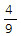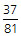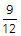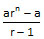# TANCET 2013 Quant Qn 1: GP

## Question

Easy Quantitative Reasoning

If a rubber ball consistently bounces back ⅔ of the height from which it is dropped, what fraction of its original height will the ball bounce after being dropped and bounced four times without being stopped?

1.2.3.4.5.Correct Answer    Choice 1.• ### Step by step solution

1. Each time the ball is dropped and it bounces back, it reaches ⅔ of the height it was dropped from.
2. After the first bounce, the ball will reach ⅔ of the height from which it was dropped - let us call it the original height.
3. After the second bounce, the ball will reach ⅔ of the height it would have reached after the first bounce.

So, at the end of the second bounce, the ball would have reached ⅔ * ⅔ of the original height =th of the original height.
4. After the third bounce, the ball will reach ⅔ of the height it would have reached after the second bounce.

So, at the end of the third bounce, the ball would have reached ⅔ * ⅔ * ⅔ =th of the original height.
5. After the fourth and last bounce, the ball will reach ⅔ of the height it would have reached after the third bounce.

So, at the end of the last bounce, the ball would have reached ⅔ * ⅔ * ⅔ * ⅔ of the original height =of the original height.

The correct answer is Choice 1.

• ### Formulae to remember in Geometric Progression

A geometric progression is such a sequence in which every subsequent term of the sequence is obtained by multiplying the preceding term with a constant number. This constant number is called the common ratio (r) of the sequence.

e.g., 2, 4, 8, 16, 32 is a geometric sequence.

The second term is obtained by multiplying the first term by 2. The third term is obtained by multiplying the second term by 2 and so on.

Formula 1: nth term of a Geometric Progression an = arn-1, where a is the first term of the sequence and r is the common ratio.

Formula 2: Sum of the first n terms of a geometric progression =Formula 3: Sum up to infinite terms of a geometric progression whose common ratio r lies between 0 and 1 i.e., S =## Online TANCET MBA CourseTry it Free!

Register in 2 easy steps and start learning in 5 minutes!